﻿ 基于混沌系统的RGB彩色图像三重置乱算法文章快速检索 高级检索
 重庆邮电大学学报(自然科学版)2018, Vol. 30Issue (6): 812-818  DOI: 10.3979/j.issn.1673-825X.2018.06.0120

### 引用本文CHEN Shanxue, TANG Yiyuan. Triple scrambling algorithm for RGB color image based on chaotic system[J]. Journal of Chongqing University of Posts and Telecommunications (Natural Science Edition), 2018, 30(6): 812-818. DOI: 10.3979/j.issn.1673-825X.2018.06.012.### 文章历史

Triple scrambling algorithm for RGB color image based on chaotic system
CHEN Shanxue, TANG YiyuanChongqing Key Laboratory of Mobile Communications Technology, Chongqing University of Posts and Telecommunications, Chongqing 400065, P. R. China
Foundation Items: The National Natural Science Foundation of China (61271260); The Chongqing Municipal Education Commission Science and Technology Research Project (KJ1400416)
Abstract: Aiming at the problem of small keyspace, insufficient sequence complexity and single encryption system in traditional chaotic encryption system, a new color image triple scrambling encryption algorithm based on chaotic system is proposed. Firstly, the Logistic chaotic sequence is used to scramble the red green blue (RGB) order of each single pixel. Then one of sequences generated from the four dimensional Rabinovich hyperchaotic system is used to scramble the image pixel position by blocking processing. And the rest three of the sequences are used to scramble and diffuse the low frequency coefficients from the image 2-D discrete wavelet transform (DWT) of each RGB channel. The algorithm makes full use of chaotic sequence features to improve the traditional chaos scrambling method, scrambles the color image pixel in all three RGB channels, and combines the diffusion process with DWT closely. Through experimental simulation, the key space, sensitivity, anti-statistical attack ability and anti-differential attack ability of the algorithm are analyzed. The results show that the algorithm can safely and effectively encrypt the image and protect the image information.
Keywords: image encryption    chaotic mapping    red green blue (RGB)    discrete wavelet transform    diffusion and scrambling
0 引言

1 混沌序列生成

Logistic混沌映射是一种常见的混沌动力系统，具有3种形式，但满映射区间小，导致迭代过程中数值变化率小，并且均匀分布特性欠佳。因此，本文采用一种改进的Logistic映射，如(1)式所示，满映射区间为xn∈[-2β, 2β]。

 ${x_{n + 1}} = 2\beta - x_n^2/\beta$ (1)

Rabinovich超混沌映射定义式为

 $\left\{ \begin{array}{l} {{\dot x}_1} = k{x_2} - a{x_1} + {x_2}{x_3}\\ {{\dot x}_2} = k{x_1} - b{x_2} - {x_1}{x_3}\\ {{\dot x}_3} = {x_1}{x_2} - d{x_3} + x_4^2\\ {{\dot x}_4} = {x_1}{x_2} + c{x_4} \end{array} \right.$ (2)

(2) 式中，a, b, c, d, k为Rabinovich超混沌系统的系统参数，当a=4, b=-0.5, c=-2.2, d=1, k=8.1时，该系统处于超混沌状态。

 $E\left( x \right) = \frac{1}{{mn}}\sum\limits_i^{mn} {{x_i}}$ (3)
 $E' = 0.16 \times {E_{\rm{R}}} + 0.50 \times {E_{\rm{G}}} + 0.34 \times {E_{\rm{B}}}$ (4)
 $\Delta = E' \times {10^{ - 10}}$ (5)

(3)—(5)式中，E'为原彩色图像各通道均值E的加权平均值(权值固定)，并将其作为密钥用于解密过程。

2 彩色图像三重加密 2.1 彩色图像RGB顺序置乱

1) 读入Logistic混沌序列Lm×n矩阵与彩色图像Im×n的三通道图像像素点值IrIgIb

2) 设Ir'Ig'Ib'为加密处理后的彩色图像的3个通道值，则根据每个l值的大小置换它所对应的像素点的RGB顺序如下。

if 0≤l≤1/6 then IgIb'；IbIg'；

elseif l≤1/3 then IrIg'；IgIr'；

elseif l≤1/2 then IrIb'；IgIr'；IbIg'；

elseif l≤2/3 then IrIg'；IgIb'；IbIr'；

elseif l≤5/6 then IrIb'；IbIr'；

elseif l≤1 then RGB顺序不变；

end；

2.2 像素位置分块置乱

1) 预处理。设定最小子块大小为Imin，中等子块大小为Imid和最大子块大小为Imax，其中，Imax能被Imin整除；对图像Im×n'做扩充处理为Imn''，使得m'和n'能被IminImidImax整除。

2) 最小块块内置乱。把图像Imn''按Imin分块后，将每小块内像素点做180°旋转，得到图像I1'。

3) 最大块块间置乱。把图像I1'按最大子块大小Imax分块并编号，每行和每列块数分别为rmax, cmax；取rmax×cmax长度的Rabinovich混沌序列，排序得到索引序列，再与分块后的图像块对应，作为分块的新编号。最后将图像块按照新编号重新排列。

4) 最大块块内置乱。取3)中的第1个图像块按Imin分块，行列块数为rm, cm，另取rm×cm个Rabinovich混沌序列将其置乱。重复操作，直到每个最大块内都被置乱得到图像I2'。

5) 中等子块块间置乱。将图像I2'按mid分块，行列块数为rmid, cmid，另取rmid×cmid个Rabinovich混沌序列将其置乱。如此，进一步得到像素位置分块置乱图像。

2.3 DWT低频系数矩阵置乱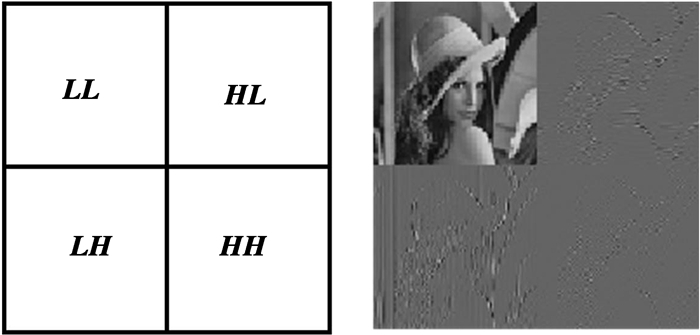图 1 2D离散小波变换示意图 Figure 1 2D discrete wavelet transformation

1) 系数置乱。将密图2的3个通道子图分别进行一级二维DWT变换后得到低频系数矩阵LLR, LLG, LLB，并转化成长度为m/2×n/2的一维序列LLR, LLG, LLB。先将剩下的3个Rabinovich超混沌序列R2R3R4进行整数求余量化，再按大小进行排序得索引序列InR, InG, InB。把Ini(i=R, G, B)分别作为各通道对应低频系数一维数组LLi(i=R, G, B)的新序号，重新排序，得到位置置乱的系数LLi'(i=R, G, B)。

2) 系数扩散。对R1, R2, R3作如下处理。

 $R'\left( i \right) = \bmod \left( {floor\left( {1\;000 \cdot {\rm{abs}}R\left( i \right)} \right),Ma{x_{LL}}} \right)$ (6)

(6) 式中：floor表示向下取整；MaxLL表示低频系数中最大值的整数部分；将得到的R'与LL'的整数部分进行按位异或，保持LL'的小数部分不变，转换成原大小矩阵获得加密后的低频系数矩阵LL"。逆变换后三通道合并得到最终的加密图像。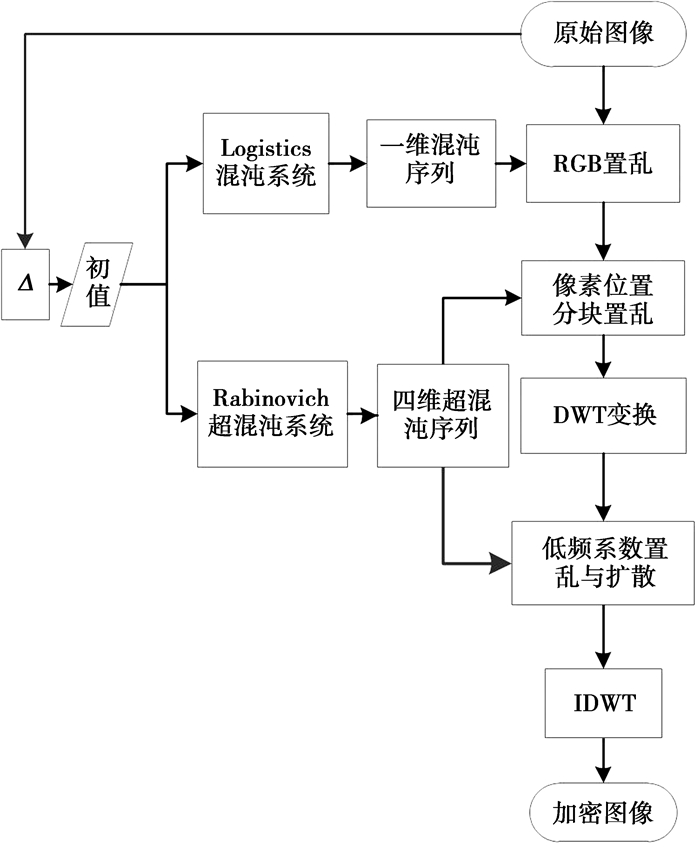图 2 算法流程图 Figure 2 Algorithm flowchart

3 实验仿真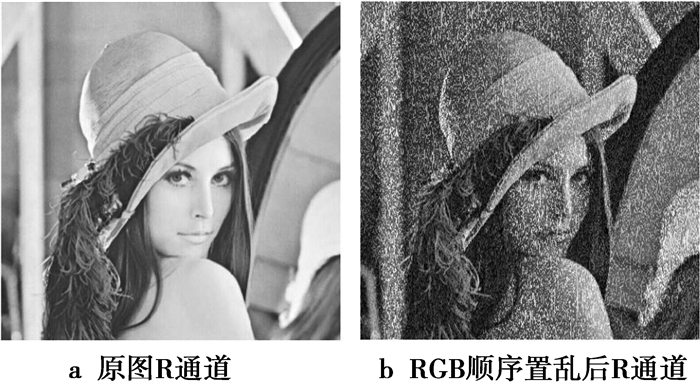图 3 RGB顺序置乱前后R通道对比图 Figure 3 Comparison diagram of each channel before and after the RGB order scrambling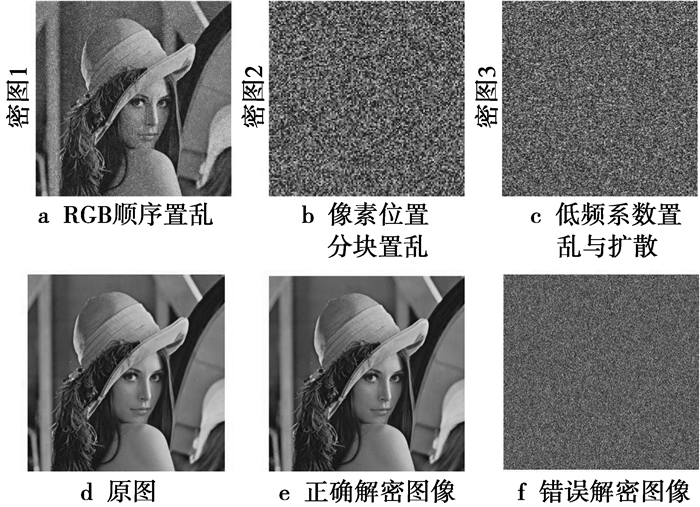图 4 加密过程图 Figure 4 Encryption process diagram

4 安全性能分析 4.1 密钥空间分析表 1 密钥空间分析 Table 1 Key space analysis
4.2 敏感度分析

4.3 抗统计攻击性能分析 4.3.1 直方图分析

Lena图像明文和密文直方图如图 5所示。图像的直方图显示了图像中像素值的分布特征。对于一个安全性高的加密算法，其密文直方图应该是均衡的。图 5中可以看出，明文直方图数据有高有低，而加密后的密文图像RGB 3个通道的直方图分布均衡。因此，攻击者不会从中获取有用信息来进行统计攻击。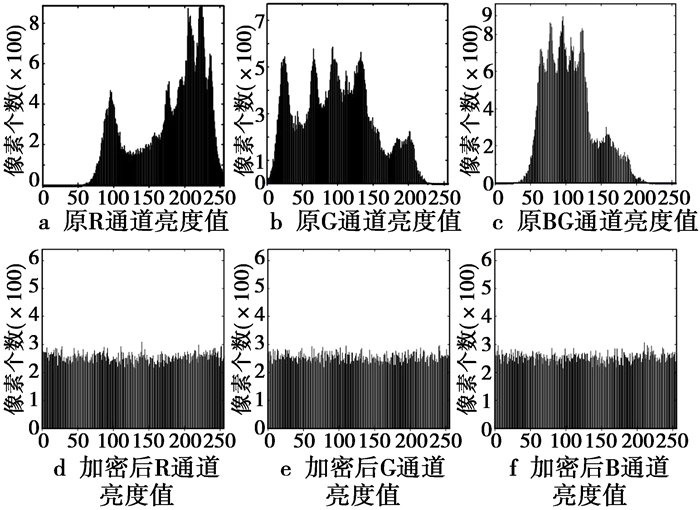图 5 直方图分析 Figure 5 Histogram analysis
4.3.2 相关性分析

 $\rho = \frac{{{\mathop{\rm cov}} \left( {x,y} \right)}}{{\sqrt {D\left( x \right)} \sqrt {D\left( y \right)} }}$ (7)
 ${\mathop{\rm cov}} \left( {x,y} \right) = \frac{1}{w}\sum\limits_i^w {\left( {{x_i} - E\left( x \right)} \right)\left( {{y_i} - E\left( y \right)} \right)}$ (8)
 $D\left( x \right) = \frac{1}{w}\sum\limits_i^w {{{\left( {{x_i} - E\left( x \right)} \right)}^2}}$ (9)表 2 RGB各通道中相邻像素相关性 Table 2 Correlation of adjacent pixels in each RGB channels

(7)—(9)式中，xy分别表示一组相邻像素点的像素值。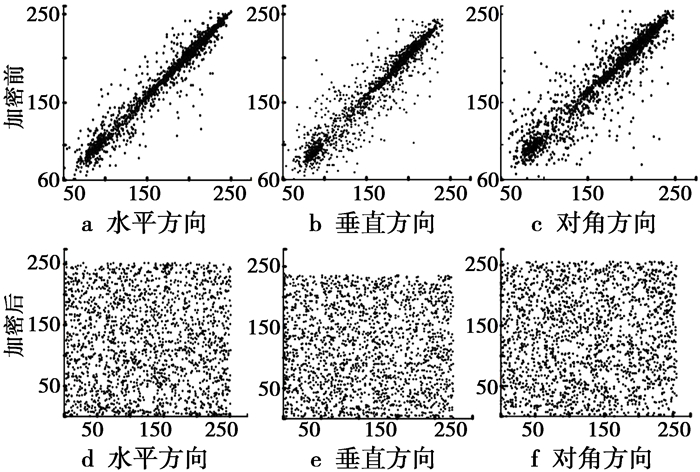图 6 R通道相关性对比 Figure 6 Comparison diagram of correlationof adjacent pixels in red channel

4.3.3 信息熵

 $H\left( m \right) = - \sum\limits_{i = 1}^{{2^n} - 1} {P\left( {{x_i}} \right){\rm{lb}}P\left( {{x_i}} \right)}$ (10)

(10) 式中，P(xi)表示各通道亮度值状态出现的概率，共2n种状态(本文中n=8)。经计算加密后图像各通道的信息熵如表 3所示，接近最大熵值8，说明该密文图像接近随机分布。表 3 信息熵 Table 3 Information entropy
4.4 抗差分攻击能力分析

 $NPCR = \frac{1}{{m \times n}}\sum\limits_{i,j} {D\left( {i,j} \right)} \times 100\%$ (11)

(11) 式中，当相同像素点坐标(i, j)的原密文图像像素值与新密文像素值一致时D(i, j)=0，否则D(i, j)=1。

 $UACI = \frac{1}{{m \times n}}\left[ {\sum\limits_{i,j} {\frac{{X\left( {i,j} \right) - X'\left( {i,j} \right)}}{{255}}} } \right] \times 100\%$ (12)表 4 密图NPCR和UACI值 Table 4 NPCR and UACI of the encrypted images
5 总结

  文昌辞, 王沁, 苗晓宁, 等. 数字图像加密综述[J]. 计算机科学, 2012, 12(12): 6-9. WENG Changci, WANG Qin, MIAO Xiaoning, et al. Digital image encryption: a survey[J]. Computer science, 2012, 12: 6-9. DOI:10.3969/j.issn.1002-137X.2012.12.002  朱从旭, 胡玉平, 孙克辉. 基于超混沌系统和密文交错扩散的图像加密新算法[J]. 电子与信息学报, 2012, 34(7): 1735-1743. ZHU Chongxu, HU Yuping, SUN Kequn. New image encryption algorithm based on hyperchaotic system and ciphertext diffusion in crisscross pattern[J]. Jounal of Electronics & Information Technology, 2012, 34(7): 1735-1743.  WU X, WANG Z. A new DWT-based lossless chaotic encryption scheme for color images[C]//2015 International Conference on Computer and Computational Sciences. Noida: IEEE Press. 2015: 211-216.  PARVEES M, SAMATH J A, RAJIK, et al.. A colour byte scrambling technique for efficient image encryption based on combined chaotic map: Image encryption using combined chaotic map[C]//2016 International Conference on Electrical, Electronics, and Optimization Techniques. Chennai: IEEE Press. 2016: 1067-1072.  WU X, ZHU B, HU Y, et al. A novel colour image encryption scheme using rectangular transform-enhanced chaotic tent maps[J]. IEEE Access, 2017(5): 6429-6436.  赵建峰, 王淑英, 李险峰, 等. 基于假分数阶激光混沌的数字图像加密研究[J]. 四川大学学报:自然科学版, 2016, 53(1): 79-86. ZHAO Jianfeng, WANG Shuying, LI Xianfeng, et al. Research on Encryption of Digital Image Based on Improper Fractional-order Laser Chaos[J]. Journal of Sichuan University:Natural Science Edition, 2016, 53(1): 79-86.  付宏睿, 史红涛, 张建刚. 基于新四翼混沌系统的复杂网络的混沌同步及其在保密通信中的应用[J]. 四川大学学报:自然科学版, 2017, 54(5): 965-970. FU Hongrui, SHI Hongtao, ZHANG Jiangang. Chaos synchronization of complex networks based on the novel four-wing chaotic system and its application in secure communication[J]. Journal of Sichuan University:Natural Science Edition, 2017, 54(5): 965-970.  JIANG H Y, FU C. An image encryption scheme based on Lorenz Chaos system[C]//2008 Fourth International Conference on Natural Computation. Jinan: IEEE Computer Society Press.2008: 600-604.  PING P, MAO Y, LV X, et al.. An image scrambling algorithm using discrete Henon map[C]//2015 IEEE International Conference on Information and Automation. Lijiang: IEEE Press. 2015: 429-432.  PRUSTY A, PATTANAIK A, MISHRA S. An image encryption & decryption approach based on pixel shuffling using Arnold Cat Map & Henon Map[C]//2013 International Conference on Advanced Computing and Communication Systems. Coimbatore: IEEE Press. 2013: 1-6.  LEI L H, BAI F M, HAN X H. New image encryption algorithm based on Logistic map and hyper-chaos[C]//2013 International Conference on Computational and Information Sciences. Shiyang: IEEE Press. 2013: 713-716.  WU X, WANG D, KURTHS J, et al. A novel lossless color image encryption scheme using 2D DWT and 6D hyperchaotic system[J]. Information Sciences, 2016(s349-350): 137-153.  王光义, 袁方. 级联混沌及其动力学特性研究[J]. 物理学报, 2013, 62(2): 103-112. WANG Yiguang, YUAN Fang. Study on cascade chaos and its dynamic characteristics[J]. Journal of Physics, 2013, 62(2): 103-112.  韩春艳, 禹思敏. 改进的logistic映射及其动力学特性[J]. 中国海洋大学学报, 2015, 45(5): 120-125. HAN Chunyan, YU Simin. Improved logistic mapping and its dynamic characteristics[J]. Periodical of Ocean University of China, 2015, 45(5): 120-125.  LIU A, LI L. Global dynamics of the stochastic Rabinovich system[J]. Nonlinear Dynamics, 2015, 81(4): 1-13.  ZHANG X, ZHU G, MA S. Remote-sensing image encryption in hybrid domains[J]. Optics Communications, 2012, 285(7): 1736-1743. DOI:10.1016/j.optcom.2011.12.023  FU C, CHEN J J, ZOU H, et al. A chaos-based digital image encryption scheme with an improved diffusion strategy[J]. Optics Express, 2012, 20(3): 2363-2378. DOI:10.1364/OE.20.002363  HUANG H, YANG S. Colour image encryption based on logistic mapping and double random-phase encoding[J]. LET Image Processing, 2017, 11(4): 211-216. DOI:10.1049/iet-ipr.2016.0552  ZHOU G, ZHANG D, LIU Y, et al. A novel image encryption algorithm based on chaos and Line map[J]. Neurocomputing, 2015(169): 150-157.  WANG X, ZHANG H L. A color image encryption with heterogeneous bit-permutation and correlated chaos[J]. Optics Communications, 2015(342): 51-60.  PATIDAR V, PAREEK N K, SUD K K. A new substitution-diffusion based image cipher using chaotic standard and logistic maps[J]. Communications in Nonlinear Science & Numerical Simulation, 2009, 14(7): 3056-3075.